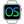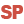Home |Sign in | EnglishHelpWLanguageWLanguage syntaxData typeAdvanced typesPointPresentationExampleWLanguage properties that can be used with the Point typeAssigning a Point variableAssigning an array of PointsComparison and serializationNotesSee alsoAdvanced typesBorderConstants for managing bordersFontPointProcedureUsing the advanced typesVariantMember
• WLanguage properties that can be used with the Point type
• Assigning a Point variable
• Assigning an array of Points
• Comparison and serialization
• Notes
WINDEVWEBDEVWINDEV MobileOthersPoint (Type of variable)
In french: Point
A Point variable is a structured type that is used to represent a position (X, Y) in 2 dimensions.
Example
MyPoint is Point
MyPoint..X = 280
MyPoint..Y = 54
Syntax

Declaring and initializing a point

<Point name> is Point
<Point name>:
Name of the Point variable to declare.
Remarks

WLanguage properties that can be used with the Point type

The initial value of a point is set to (0,0).
The following properties can be used to handle a Point variable:
Property name Effect X X coordinate of the point. This coordinate corresponds to a signed 4-byte integer. Y Y coordinate of the point. This coordinate corresponds to a signed 4-byte integer.

Assigning a Point variable

A Point variable can be assigned with:
• another point.
For example:
MyPoint is Point
MyPoint..X = 280
MyPoint..Y = 54
MyPoint1 is Point = MyPoint
• an array of two values that can be converted into integer.
For example:
MyPoint is Point
MyPoint = [280, 54]

Assigning an array of Points

An array of Points can be assigned with:
• an array of values containing points.
For example:
ArrayOfPoints is array of Points
ArrayOfPoints = [MyPoint1,MyPoint2]
• an array of two values that can be converted into integer.
For example:
ArrayOfPoints is array of Points
ArrayOfPoints = [[1,2],[3,4]]

Comparison and serialization

• Comparison: The comparison operators = and <> are available.
• Serialization: The binary, XML and JSON serializations are available.
Caution: During an XML serialization, xmlAttribute cannot be used on a Point class member or on a Point structure member.

Notes

• A Point variable can be stored in a variant.
• A Point variable is never null:
• The "PointVariable = NULL" condition always returns False.
• The "PointVariable <> NULL" condition always returns True.
• You cannot reference a Point variable.
The "PointVariable2 <- PointVariable1" syntax triggers an error.
• You cannot force the copy of a Point variable.
The "PointVariable2 <= PointVariable1" syntax triggers an error.
• You have the ability to add and subtract two Point variables.
• The Point variables can be passed to the system APIs.
Minimum version required
• Version 21